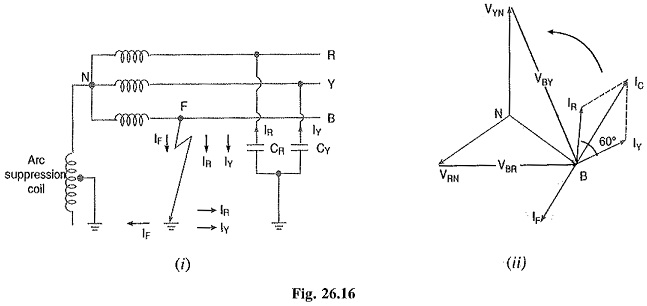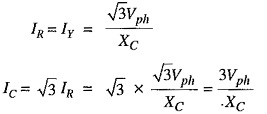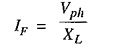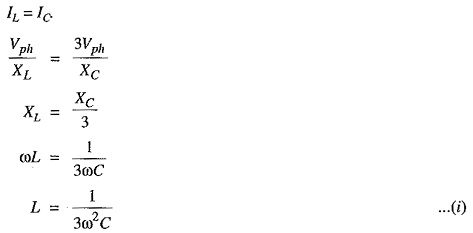## Resonant Grounding or Arc Suppression Coil Grounding:

We have seen that capacitive currents are responsible for producing arcing grounds. These capaci­tive currents flow because capacitance exists between each line and earth. If inductance L of appro­priate value is connected in parallel with the capacitance of the system, the fault current IF flowing through L will be in phase opposition to the capacitive current IC of the system. If L is so adjusted that I= IC then resultant current in the fault will be zero. This condition is known as Resonant Grounding.

When the value of L of arc suppression coil is such that the fault current IF exactly balances the capacitive current IC, it is called Resonant Grounding.

Circuit details: An arc suppression coil (also called Peterson coil) is an iron-cored coil connected between the neutral and earth as shown in Fig. 26.160). The reactor is provided with tapping to change the inductance of the coil. By adjusting the tappings on the boil, the coil can be tuned with the capacitance of the system i.e. resonant grounding can be achieved.Operation: Fig. 26.160) shows the 3-phase system employing Peterson coil grounding. Suppose line to ground fault occurs in the line B at point F. The fault current IF and capacitive currents IR and IY will flow as shown in Fig. 26.160). Note that IF flows through the Peterson coil (or Arc suppression coil) to neutral and back through the fault. The total capacitive current IC is the phasor sum of IR and IY as shown in phasor diagram in Fig. 26.16(11). The voltage of the faulty phase is applied across the arc suppression coil. Therefore, fault current IF lags the faulty phase voltage by 90°. The current IF is in phase opposition to capacitive current IC [See Fig. 26.16(ii)]. By adjusting the tappings on the Peterson coil, the resultant current in the fault can be reduced, If inductance of the coil is so adjusted that IL = IC then resultant current in the fault will be zero.

Value of L for resonant grounding: For resonant grounding, the system behaves as an un­grounded neutral system. Therefore, full line voltage appears across capacitors CR and CY.Here, XC is the line to ground capacitive reactance.Here, XL is the inductive reactance of the arc suppression coil.

For resonant grounding,Exp. (i) gives the value of inductance L of the arc suppression coil for resonant grounding.

#### The Peterson coil grounding has the following advantages:

• The Peterson coil is completely effective in preventing any damage by an arcing ground.
• The Peterson coil has the advantages of ungrounded neutral system.

#### The Peterson coil grounding has the following disadvantages :

• Due to varying operational conditions, the capacitance of the network changes from time to time. Therefore, inductance L of Peterson coil requires readjustment.
• The lines should be transposed.
Scroll to Top